numpy.polynomial.chebyshev.chebgauss¶

numpy.polynomial.chebyshev.chebgauss(deg)[source]

Computes the sample points and weights for Gauss-Chebyshev quadrature. These sample points and weights will correctly integrate polynomials of degree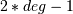or less over the interval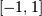with the weight function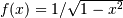.

Parameters : deg : int Number of sample points and weights. It must be >= 1. x : ndarray 1-D ndarray containing the sample points. y : ndarray 1-D ndarray containing the weights.

Notes

New in version 1.7.0.

The results have only been tested up to degree 100, higher degrees may be problematic. For Gauss-Chebyshev there are closed form solutions for the sample points and weights. If n = deg, then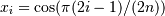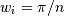Previous topic

numpy.polynomial.chebyshev.chebpow

Next topic

numpy.polynomial.chebyshev.chebweight